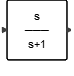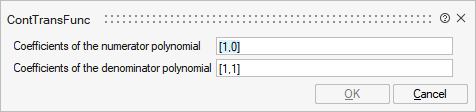# ContTransFunc

This block implements a continuous-time linear system that is defined by its rational transfer function (Numerator/Denominator). The transfer function must be proper meaning that the degree of numerator must not exceed that of the denominator. In the MIMO case, the numerator and denominator must be defined as cells (see OML tf2ssc function for details).## Library

Activate/Dynamical

## Description

This block realizes the transfer function of a continuous linear system.

This block implements a continuous-time linear system represented by its rational transfer function (Numerator/Denominator). The transfer function must be proper, meaning that the degree of its numerator must not exceed that of its denominator. In the SISO case, the input and output may be of arbitrary but equal size, in which case the SISO system is applied element-wise.

The block has one input and one output.

## ParametersNameLabelDescriptionData TypeValid Values

num

Coefficients of the numerator polynomial

An mxn matrix where m is the size of the output, and n is the order of the numerator polynomial. Alternatively a cell may be used in the MIMO case.

den

Coefficients of the denominator polynomial

This parameter sets the denominator of the transfer function. This must be a polynomial. Alternatively a cell is used in the MIMO case.

## Ports

NameTypeDescriptionIO TypeNumber

Port 1

explicit

output

1

Port 2

explicit

input

1

NameValueDescription

always active

yes

direct-feedthrough

no

no, unless the order of numerator is equal to the order of denominator.

zero-crossing

no

mode

no

continuous-time state

yes

discrete-time state

no